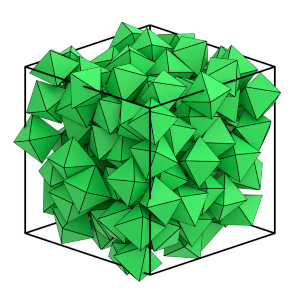# Compressing the System#

## Overview#

### Questions#

• How do I compress the system to a target density?

• What is a volume fraction?

### Objectives#

• Show how to compute the volume fraction of a system.

• Explain how how an Updater is an operation that modifies the system when its Trigger returns True.

• Demonstrate using the QuickCompress updater to achieve a target volume fraction.

• Demonstrate using the MoveSize tuner to adjust the trial move size.

## Boilerplate code#

:

import copy
import math

import hoomd


The render function in the next (hidden) cell will render a snapshot using fresnel. Find the source in the hoomd-examples repository.

## Volume fraction#

Self-assembly in hard particle systems typically occurs at a volume fraction above 0.5. The volume fraction is the ratio of the volume occupied by the particles to the volume of the periodic box.

So far, this tutorial as randomized a system of N octahedra in a box with a very low volume fraction and stored that in random.gsd. Initialize a Simulation with this configuration and see what volume fraction it is at:

:

cpu = hoomd.device.CPU()
simulation = hoomd.Simulation(device=cpu, seed=20)
simulation.create_state_from_gsd(filename='random.gsd')


Compute the volume of the octahedron:

:

a = math.sqrt(2) / 2
V_particle = 1 / 3 * math.sqrt(2) * a**3


Compute the volume fraction:

:

initial_volume_fraction = (simulation.state.N_particles * V_particle
/ simulation.state.box.volume)
print(initial_volume_fraction)

0.05715592589579699


As you can see, this volume fraction is very low and the box volume needs to be significantly reduced to achieve a volume fraction above 0.5.

Use HPMC to move particles into non-overlapping configurations while you compress the system. Set up the HPMC integrator for the octahedron simulations:

:

mc = hoomd.hpmc.integrate.ConvexPolyhedron()
mc.shape['octahedron'] = dict(vertices=[
(-0.5, 0, 0),
(0.5, 0, 0),
(0, -0.5, 0),
(0, 0.5, 0),
(0, 0, -0.5),
(0, 0, 0.5),
])
simulation.operations.integrator = mc


## The QuickCompress updater#

An Updater is a type of operation in HOOMD-blue that makes changes to the state. To use an Updater, first instantiate the object, assign a Trigger, and add it to the Simulation. Simulation will apply the Updater on time steps where the Trigger returns True. The Periodic trigger returns True every period steps.

QuickCompress is an Updater that works with HPMC to quickly compress the box to a target volume. When triggered, QuickCompress reduces the box volume by a scale factor, while allowing slight overlaps between the particles. It then waits for the translation and rotation trial moves to remove these overlaps before it reduces the volume again. This process temporarily produces invalid system configurations, but is much quicker than a process that does not allow temporary overlaps.

Compute the final box size with a volume fraction above 0.5 and configure a QuickCompress to trigger every 10 time steps.

:

initial_box = simulation.state.box
final_box = hoomd.Box.from_box(initial_box)
final_volume_fraction = 0.57
final_box.volume = simulation.state.N_particles * V_particle / final_volume_fraction
compress = hoomd.hpmc.update.QuickCompress(trigger=hoomd.trigger.Periodic(10),
target_box=final_box)


Add the Updater to the Simulation:

:

simulation.operations.updaters.append(compress)


## The MoveSize tuner#

A Tuner is another type of operation. Tuners make changes to other operations to improve performance. In HPMC, the translation and rotation trial move sizes have a huge performance impact. When the move size is too small it takes many time steps to make appreciable changes to the system. When the move size is too large very few moves are accepted and it again takes many time steps to make appreciable changes. The system makes the most progress at moderate move sizes and, in most cases, the optimal acceptance ratio is 20%. The MoveSize tuner monitors the acceptance ratio and adjusts d and a to achieve the target.

The optimal move size depends on the density of the system. QuickCompress changes the density rapidly during compression, so use the MoveSize tuner to adjust the move sizes regularly:

:

periodic = hoomd.trigger.Periodic(10)
tune = hoomd.hpmc.tune.MoveSize.scale_solver(moves=['a', 'd'],
target=0.2,
trigger=periodic,
max_translation_move=0.2,
max_rotation_move=0.2)
simulation.operations.tuners.append(tune)


## Run until complete#

When the QuickCompress updater achieves the target box size and there are no overlaps between particles, the compression process is complete. The number of time steps needed to achieve this varies based on parameters. Check the compress.complete property regularly and stop running the simulation when the compression completes:

:

while not compress.complete and simulation.timestep < 1e6:
simulation.run(1000)


The simulation.timestep < 1e6 check ensures that this while loop will not waste resources in cases where the compression will never complete. The loop should complete before that point:

:

simulation.timestep

:

13000


Check to see if the compression completed successfully:

:

if not compress.complete:
raise RuntimeError("Compression failed to complete")


The MoveSize tuner should have adjusted the move sizes to relatively small values at the final density:

:

mc.a['octahedron']

:

0.0573165403351847

:

mc.d['octahedron']

:

0.031253275743820426


Now that the compression is complete, the particles are much closer together and not overlapping, but still arranged randomly.

:

render(simulation.state.get_snapshot())

:Save the final configuration to a GSD file for use in the next stage of the simulation:

:

hoomd.write.GSD.write(state=simulation.state,
mode='xb',
filename='compressed.gsd')